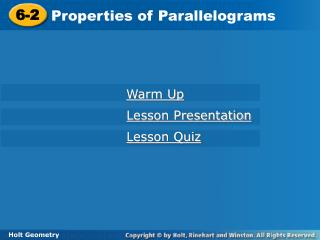DownloadDownload Presentation6-2

# 6-2

Download Presentation## 6-2

- - - - - - - - - - - - - - - - - - - - - - - - - - - E N D - - - - - - - - - - - - - - - - - - - - - - - - - - -
##### Presentation Transcript

1. 6-2 Properties of Parallelograms Warm Up Lesson Presentation Lesson Quiz Holt Geometry

2. Warm Up Find the value of each variable. 1.x2.y 3.z 2 18 4

3. Objectives Prove and apply properties of parallelograms. Use properties of parallelograms to solve problems.

4. Vocabulary parallelogram

5. Any polygon with four sides is a quadrilateral. However, some quadrilaterals have special properties. These special quadrilaterals are given their own names.

6. Helpful Hint Opposite sides of a quadrilateral do not share a vertex. Opposite angles do not share a side.

7. A quadrilateral with two pairs of parallel sides is a parallelogram. To write the name of a parallelogram, you use the symbol .

8. In CDEF, DE = 74 mm, DG = 31 mm, and mFCD = 42°. Find CF. opp. sides Example 1A: Properties of Parallelograms CF = DE Def. of segs. CF = 74 mm Substitute 74 for DE.

9. In CDEF, DE = 74 mm, DG = 31 mm, and mFCD = 42°. Find mEFC. cons. s supp. Example 1B: Properties of Parallelograms mEFC + mFCD = 180° mEFC + 42= 180 Substitute 42 for mFCD. mEFC = 138° Subtract 42 from both sides.

10. In CDEF, DE = 74 mm, DG = 31 mm, and mFCD = 42°. Find DF. diags. bisect each other. Example 1C: Properties of Parallelograms DF = 2DG DF = 2(31) Substitute 31 for DG. DF = 62 Simplify.

11. opp. sides Check It Out! Example 1a In KLMN, LM = 28 in., LN = 26 in., and mLKN = 74°. Find KN. LM = KN Def. of segs. LM = 28 in. Substitute 28 for DE.

12. opp. s  Check It Out! Example 1b In KLMN, LM = 28 in., LN = 26 in., and mLKN = 74°. Find mNML. NML  LKN mNML = mLKN Def. of  s. mNML = 74° Substitute 74° for mLKN. Def. of angles.

13. diags. bisect each other. Check It Out! Example 1c In KLMN, LM = 28 in., LN = 26 in., and mLKN = 74°. Find LO. LN = 2LO 26 = 2LO Substitute 26 for LN. LO = 13 in. Simplify.

14. opp. s  Example 2A: Using Properties of Parallelograms to Find Measures WXYZ is a parallelogram. Find YZ. YZ = XW Def. of  segs. 8a – 4 = 6a + 10 Substitute the given values. Subtract 6a from both sides and add 4 to both sides. 2a = 14 a = 7 Divide both sides by 2. YZ = 8a – 4 = 8(7) – 4 = 52

15. cons. s supp. Example 2B: Using Properties of Parallelograms to Find Measures WXYZ is a parallelogram. Find mZ. mZ + mW = 180° (9b + 2)+ (18b –11) = 180 Substitute the given values. Combine like terms. 27b – 9 = 180 27b = 189 b = 7 Divide by 27. mZ = (9b + 2)° = [9(7) + 2]° = 65°

16. diags. bisect each other. Check It Out! Example 2a EFGH is a parallelogram. Find JG. EJ = JG Def. of  segs. 3w = w + 8 Substitute. 2w = 8 Simplify. w = 4 Divide both sides by 2. JG = w + 8 = 4 + 8 = 12

17. diags. bisect each other. Check It Out! Example 2b EFGH is a parallelogram. Find FH. FJ = JH Def. of  segs. 4z – 9 = 2z Substitute. 2z = 9 Simplify. z = 4.5 Divide both sides by 2. FH = (4z – 9) + (2z) = 4(4.5) – 9 + 2(4.5) = 18

18. Example 4A: Using Properties of Parallelograms in a Proof Write a two-column proof. Given: ABCD is a parallelogram. Prove:∆AEB∆CED

19. 3. diags. bisect each other 2. opp. sides  Example 4A Continued Proof: 1. ABCD is a parallelogram 1. Given 4. SSS Steps 2, 3

20. Example 4B: Using Properties of Parallelograms in a Proof Write a two-column proof. Given: GHJN and JKLM are parallelograms. H and M are collinear. N and K are collinear. Prove:H M

21. 2.H and HJN are supp. M and MJK are supp. 2. cons. s supp. Example 4B Continued Proof: 1.GHJN and JKLM are parallelograms. 1. Given 3.HJN  MJK 3. Vert. s Thm. 4.H  M 4. Supps. Thm.

22. Check It Out! Example 4 Write a two-column proof. Given: GHJN and JKLM are parallelograms. H and M are collinear. N and K are collinear. Prove: N  K

23. 2.N and HJN are supp. K and MJK are supp. 2. cons. s supp. Check It Out! Example 4 Continued Proof: 1.GHJN and JKLM are parallelograms. 1. Given 3.HJN  MJK 3. Vert. s Thm. 4.N  K 4. Supps. Thm.

24. Lesson Quiz: Part I In PNWL, NW = 12, PM = 9, and mWLP = 144°. Find each measure. 1.PW2. mPNW 18 144°

25. Lesson Quiz: Part II QRST is a parallelogram. Find each measure. 2.TQ3. mT 71° 28

26. 1.RSTU is a parallelogram. 1. Given 3.R  T 4. ∆RSU∆TUS 4. SAS 2. cons. s  3. opp. s  Lesson Quiz: Part IV 6. Write a two-column proof. Given:RSTU is a parallelogram. Prove: ∆RSU∆TUS